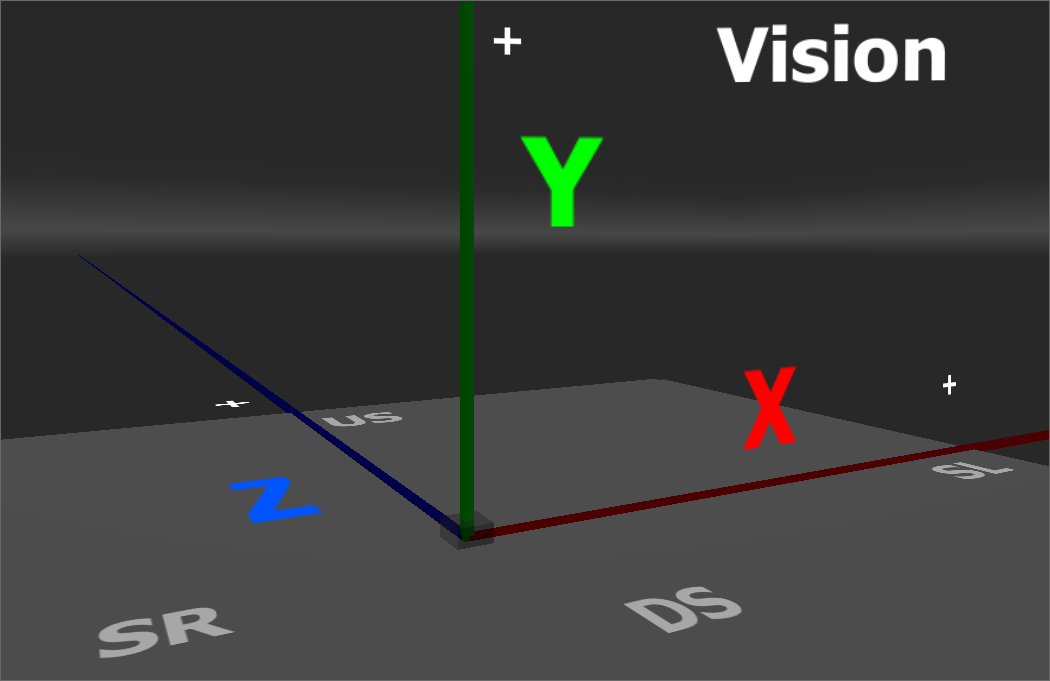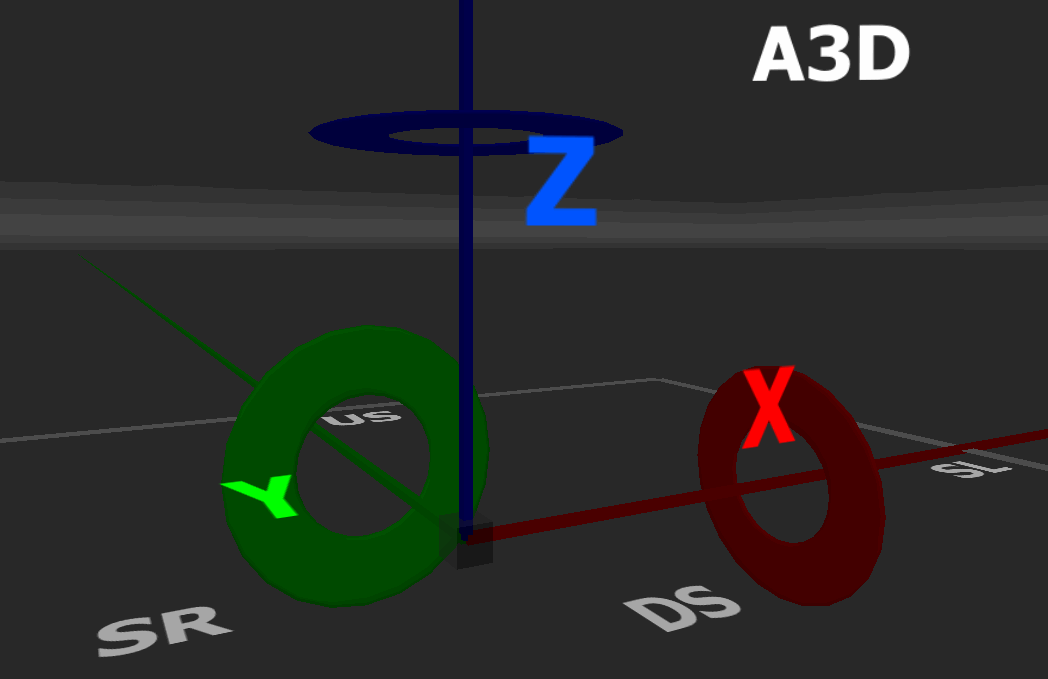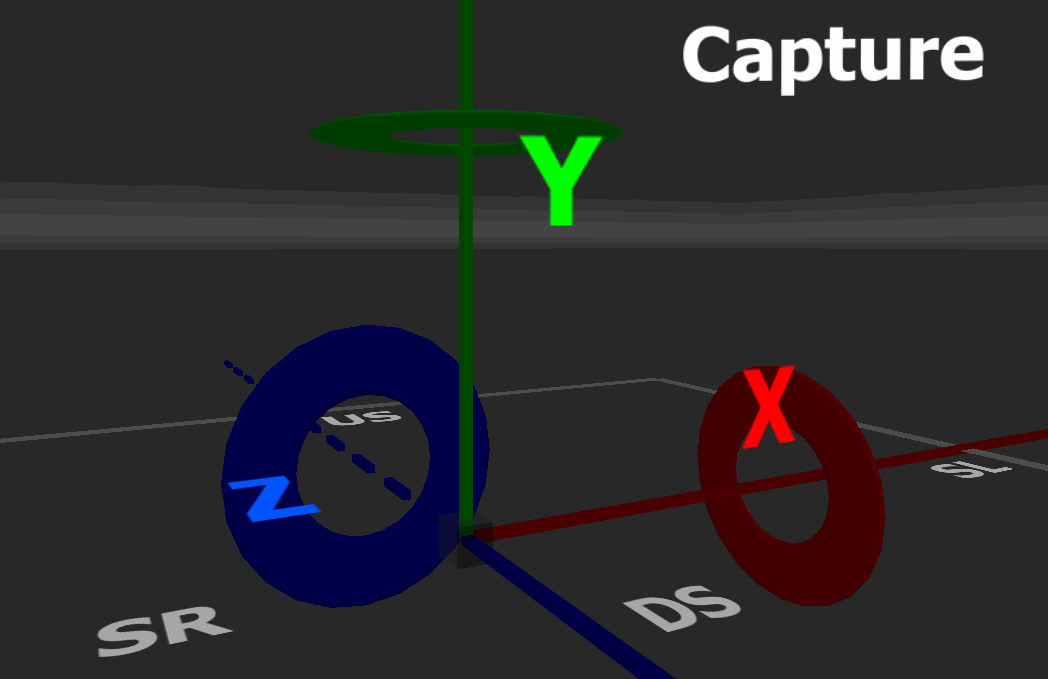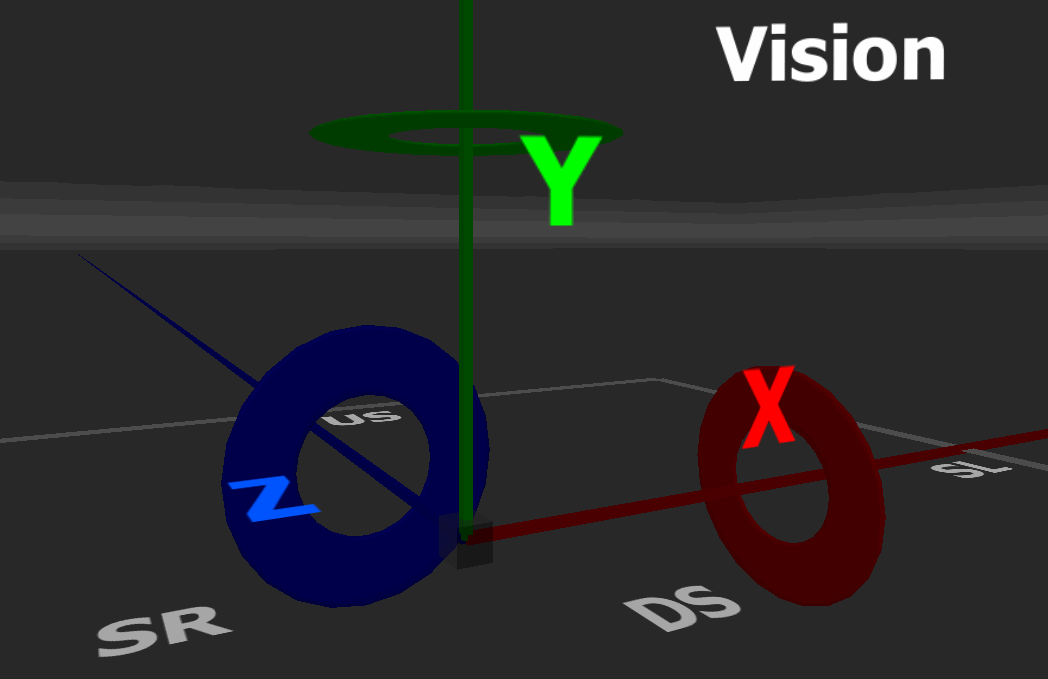/*]]>*/

# Augment3d Coordinate System Compared with Other ProgramsNote: AutoCAD, Blender, SketchUp, and Vectorworks all use the same coordinate system as Augment3d and are omitted from this comparison for brevity.

## Vision

Coordinate System GraphicAugment3dCaptureVison
Coordinate System Description Z-Up Positive Y Y-Up Negative Z Y-Up Positive Z
Coordinate Conversion to Augment3d N/A
Capture = A3d
X = X
Y = Z
Z = -Y
Vision = A3d
X = X
Y = Z
Z = Y
Coordinate Conversion to Capture
A3d = Capture
X = X
Y = -Z
Z = Y
N/A
Vision = Capture
X = X
Y = Y
Z = -Z
Coordinate Conversion to Vision
A3d = Vision
X = X
Y = Z
Z = Y
Capture = Vision
X = X
Y = Y
Z = -Z
N/A

## Vision

Rotation System GraphicAugment3dCaptureVision

Rotation System Description

(From Origin Looking Towards Positive Values on Each Axis)

Positive Rotation Clockwise Around All Axes Positive Rotation Clockwise Around All Axes

Positive Rotation Counter-Clockwise Around All Axes

Rotation Conversion to Augment3d N/A
Capture = A3d
X = X
Y = Z
Z = -Y
Vision = A3d
X = -X
Y = -Z
Z = -Y
Rotation Conversion to Capture
A3d = Capture
X = X
Y = -Z
Z = Y
N/A
Vision = Capture
X = -X
Y = -Y
Z = Z
Rotation Conversion to Vision
A3d = Vision
X = -X
Y = -Z
Z = -Y
Capture = Vision
X = -X
Y = -Y
Z = Z
N/A